Question

A. Preparation of Sodium Hydroxide Solution g g mass of Erlenmeyer flask + KHP 00 09...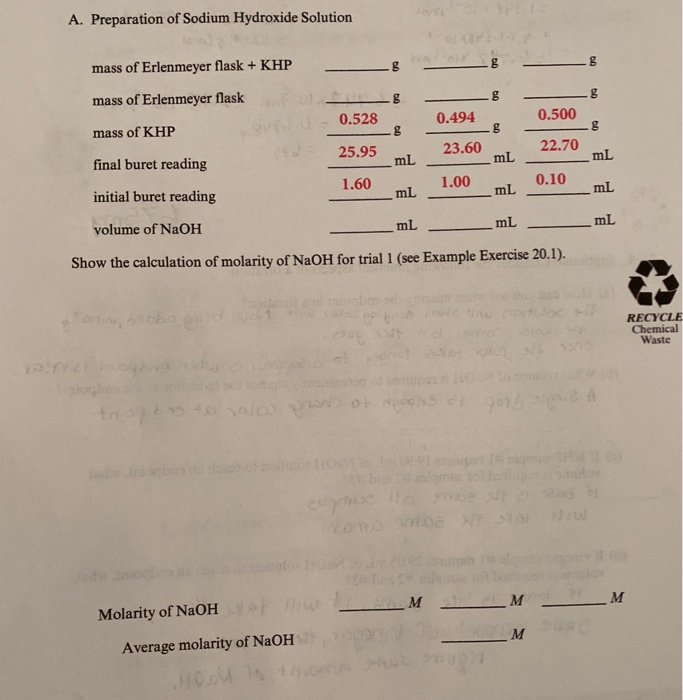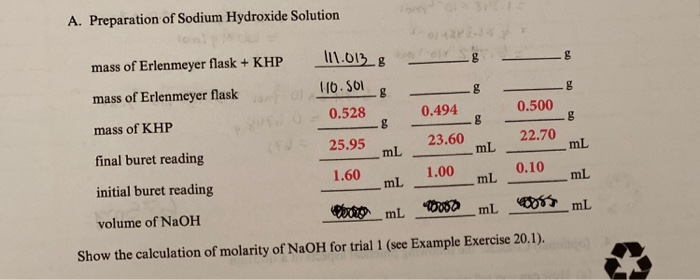A. Preparation of Sodium Hydroxide Solution g g mass of Erlenmeyer flask + KHP 00 09 mass of Erlenmeyer flask 0.494 0.500 mass of KHP LES 0.528 g 25.95 mL g 23.60 22.70 mL mL final buret reading 1.60 1.00 0.10 mL mL mL initial buret reading mL mL mL volume of NaOH Show the calculation of molarity of NaOH for trial 1 (see Example Exercise 20.1). 305 RECYCLE Chemical Waste volo Wo NON Omer _M M M Molarity of NaOH M Average molarity of NaOH
A. Preparation of Sodium Hydroxide Solution 111.0138 8 mass of Erlenmeyer flask + KHP g 8 g mass of Erlenmeyer flask 116. S01 0.528 0.494 0.500 g g mass of KHP 25.95 23.60 22.70 mL mL mL final buret reading 1.60 1.00 0.10 mL mL mL initial buret reading 10000 mL mL mL volume of NaOH Show the calculation of molarity of NaOH for trial 1 (see Example Exercise 20.1).

We need at least 10 more requests to produce the answer.

0 / 10 have requested this problem solution

The more requests, the faster the answer.

All students who have requested the answer will be notified once they are available.

Earn Coins

Coins can be redeemed for fabulous gifts.

Similar Homework Help Questions
• DATA TABLE A. Preparation of Sodium Hydroxide Solution mass of Erlenmeyer flask + KHP 2012g 76-16 71.67 g 75.96 g 0.5& 05g 11,4 mL 12.3 mL mLmL 2.3 mL mass of Erlenmeyer flask mass of KHP fi...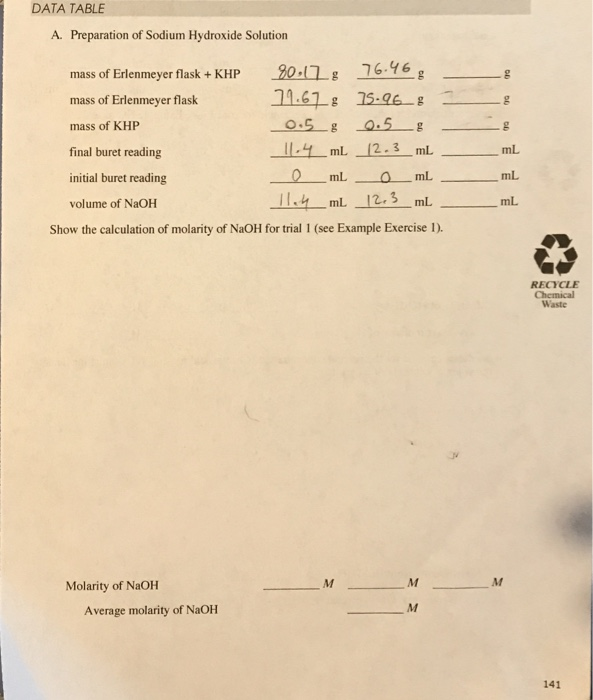DATA TABLE A. Preparation of Sodium Hydroxide Solution mass of Erlenmeyer flask + KHP 2012g 76-16 71.67 g 75.96 g 0.5& 05g 11,4 mL 12.3 mL mLmL 2.3 mL mass of Erlenmeyer flask mass of KHP final buret reading initial buret reading volume of NaOH g mL mL Show the calculation of molarity of NaOH for trial 1 (see Example Exercise RECYCLE Waste Molarity of NaOH Average molarity of NaOH 141 Analysis of Vinegar UNKNOWN # B. Titration of Acetic...

• A. Standardization of Sodium Hydroxide (NaOH) Solution Mass of bottle + KHP Mass of bottle Mass...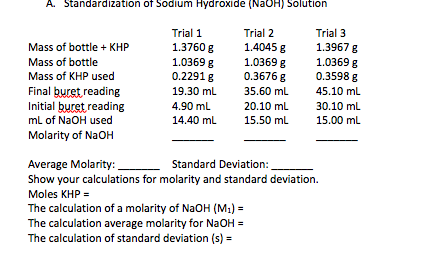A. Standardization of Sodium Hydroxide (NaOH) Solution Mass of bottle + KHP Mass of bottle Mass of KHP used Final buret reading Initial buret reading mL of NaOH used Molarity of NaOH Trial 1 1.3760 g 1.0369 g 0.2291 8 19.30 ml 4.90 mL 14.40 ml Trial 2 1.40458 1.0369 g 0.36768 35.60 mL 20.10 ml 15.50 ml Trial 3 1.39678 1.0369 g 0.3598 g 45.10 mL 30.10 ml 15.00 ml Average Molarity: Standard Deviation: Show your calculations for molarity...

• What molarity of sodium hydroxide solution is used to react 0.511 grams of KHP, if the...

What molarity of sodium hydroxide solution is used to react 0.511 grams of KHP, if the initial buret reading for the addition of sodium hyrdoxide is 0.4mL and the final reading is 12.7 mL? The molar mass of KHP is 204.2 g/mol. The reaction is KHP + NaOH --> KNaP +H2O

• Mass of empty flask (9) Mass of flask + KHP (9) Mass of KHP (g) TRIAL 1 L TRIAL 2 TRIAL 3 94.560g 89.0050 89 7357 9...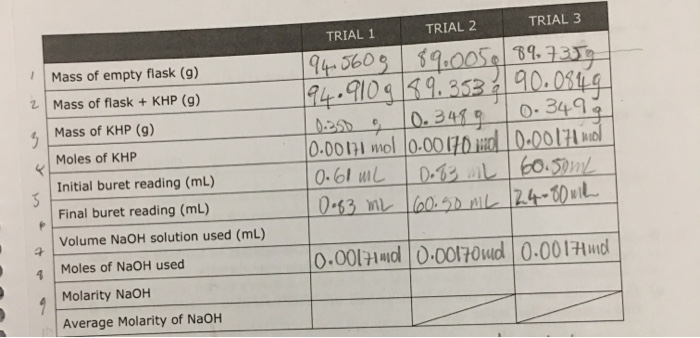Mass of empty flask (9) Mass of flask + KHP (9) Mass of KHP (g) TRIAL 1 L TRIAL 2 TRIAL 3 94.560g 89.0050 89 7357 94.9109 89.353 : 90.0949 0.250 0.3489 0.3499 0.00171 mol 0.00170.10 0.00171 wol 10.60 mL 0.83 L 60.50mL 10.83 mL 60.50 ML 124-80 with Moles of KHP Initial buret reading (mL) Final buret reading (mL) Volume NaOH solution used (ml) Moles of NaOH used Molarity NaOH Average Molarity of NaOH 0.00171md 0.00170 ud 0.0017 md

• Titration of Acids and Bases Trial 3 A. Standardization of Sodium Hydroxide (NaOH) Solution Trial I...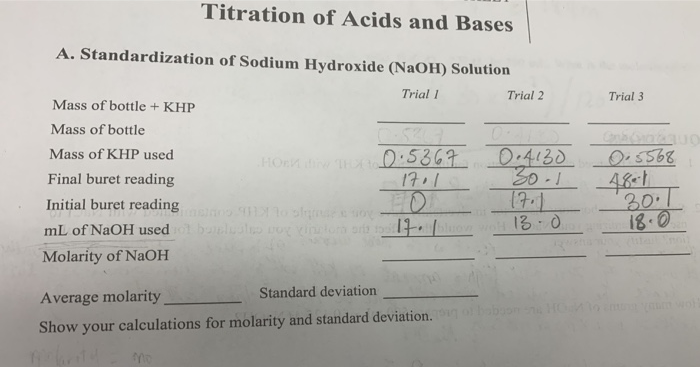Titration of Acids and Bases Trial 3 A. Standardization of Sodium Hydroxide (NaOH) Solution Trial I Trial 2 Mass of bottle + KHP Mass of bottle Mass of KHP used Horn 0.5367 0.4130 Final buret reading Initial buret reading 17.1 mL of NaOH used ol bullosa Gluon 13 .0 Molarity of NaOH 0.8568 17. 301 18.0 Average molarity Standard deviation Show your calculations for molarity and standard deviation.

• Preparation of Sodium Hydroxide Titrant: Prepare 500 mL of a 0.1 M sodium hydroxide solution by...

Preparation of Sodium Hydroxide Titrant: Prepare 500 mL of a 0.1 M sodium hydroxide solution by dilution of a 1 M sodium hydroxide stock reagent or from solid NaOH. This dilution need only be performed to 5% accuracy, that is, using a graduated cylinder and an Erlenmeyer flask. Store the diluted solution in a polyethylene bottle. Standardize the diluted solution against dry, solid KHP (potassium hydrogen phthalate) using phenolphthalein as an indicator and label the bottle with the concentration of...

• Determination of the Molarity of a Sodium Hydroxide Solution Trial 1 Trial 2 Trial 3 Weight...

Determination of the Molarity of a Sodium Hydroxide Solution Trial 1 Trial 2 Trial 3 Weight of KHP: 0.705g 0.702g 0.705g Starting Buret Reading: 4.00 mL 4.00 mL 4.00 mL Ending Buret Reading: 39.20 mL 36.70 mL 37.50 mL mL of Titrant Used: 35.2 mL 32.7 mL 33.5 mL mmole of KHP: mmole of NaOH: Molarity of NaOH solution: Average Molarity:       Fill in the table and show work please.

• Needing help on how to fins the mass of KHP. I can solve for the percent...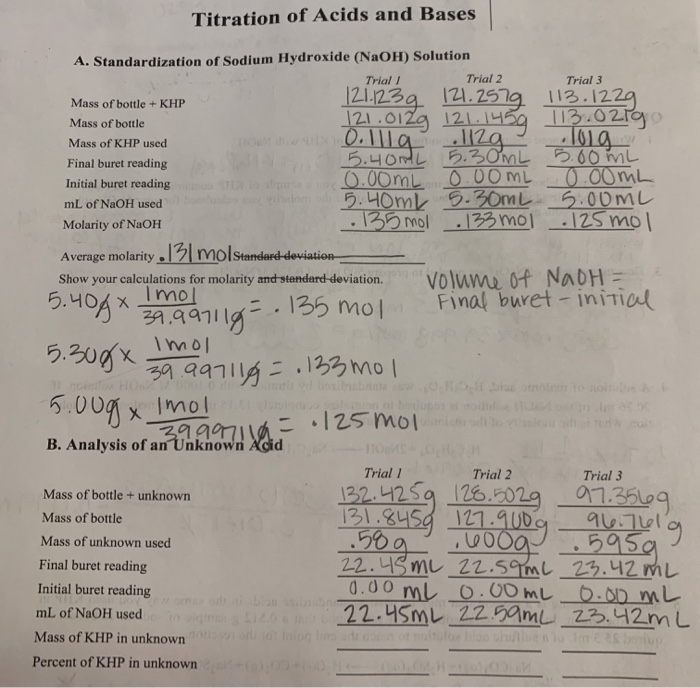Needing help on how to fins the mass of KHP. I can solve for the percent from there. Titration of Acids and Bases A. Standardization of Sodium Hydroxide (NaOH) Solution Trial Trial 2 Trial 3 Mass of bottle + KHP 121.123g 121.2579 113.1229 Mass of bottle 121.012g 121.1459 113.0279 Mass of KHP used 0.llla 112g lola Final buret reading 5.400L 5. 30ML 5.00mL Initial buret reading 0.00ML 0.00ML 0.00ML mL of NaOH used 5.40ml 5.30mL 500ML Molarity of NaOH -...

• Questions: A. From the standardization data, calculate the molarity of the sodium hydroxide solution for each...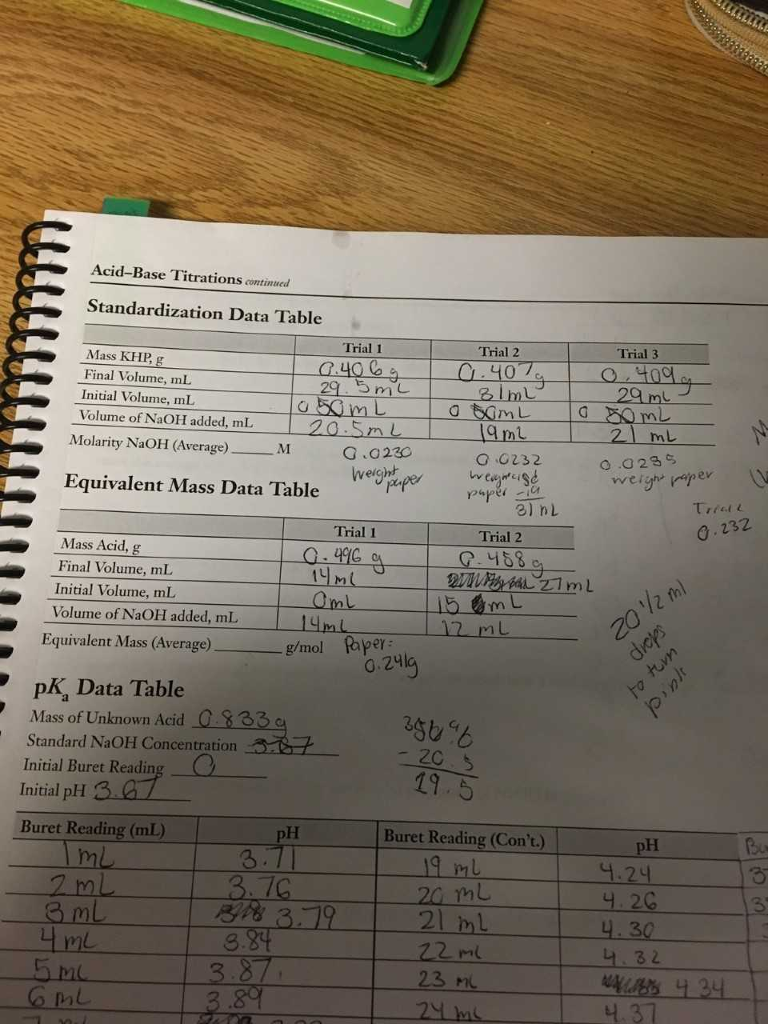Questions: A. From the standardization data, calculate the molarity of the sodium hydroxide solution for each trail. Average the values and enter the average in the Standardization Data Table. B. From the equivalent mass data, calculate the equivalent mass of the unknown acid for each trial. Average the values and enter the average in the Equivalent Mass Data Table. Acid-Base Titrations continued Standardization Data Table Trial 1 Trial 2 Trial 3 Mass KHP Final Volume, mL Initial Volume, ml Volume...

• Can I get some help filling the rest out? A. PREPARATION OF SODIUM HYDROXIDE SOLUTION Mass...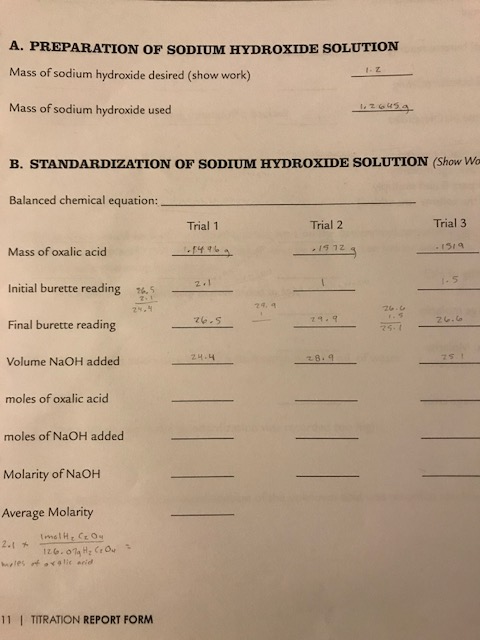Can I get some help filling the rest out? A. PREPARATION OF SODIUM HYDROXIDE SOLUTION Mass of sodium hydroxide desired (show work) Mass of sodium hydroxide used B. STANDARDIZATION OF SODIUM HYDROXIDE SOLUTION (Show Wa Balanced chemical equation Trial 3 Trial 1 Trial 2 "เราจ Mass of oxalic acid 2.1 Initial burette reading to e, 5 29.9 Final burette reading 75 1 Volume NaOH added 28.4 moles of oxalic acid moles of NaOH added Molarity of NaOH Average Molarity 2.1...